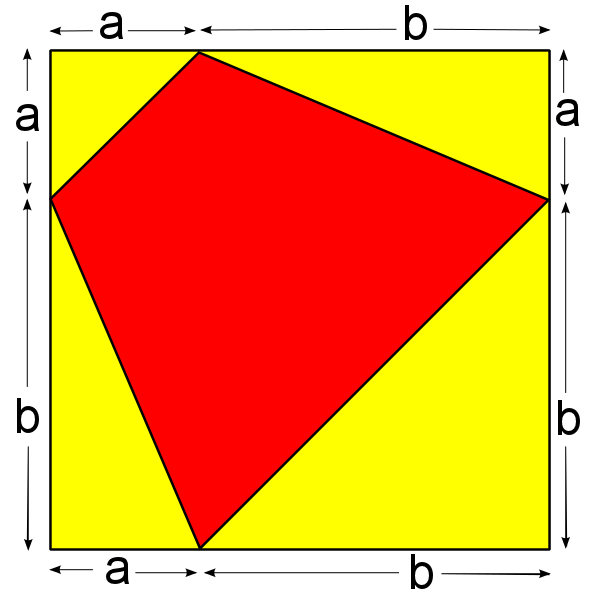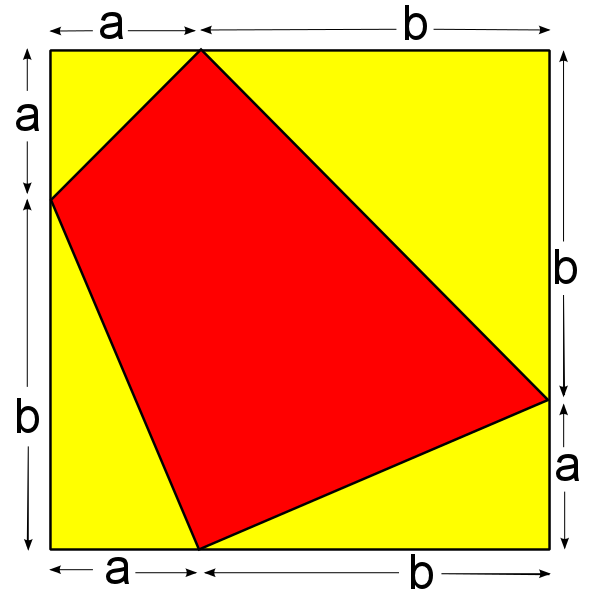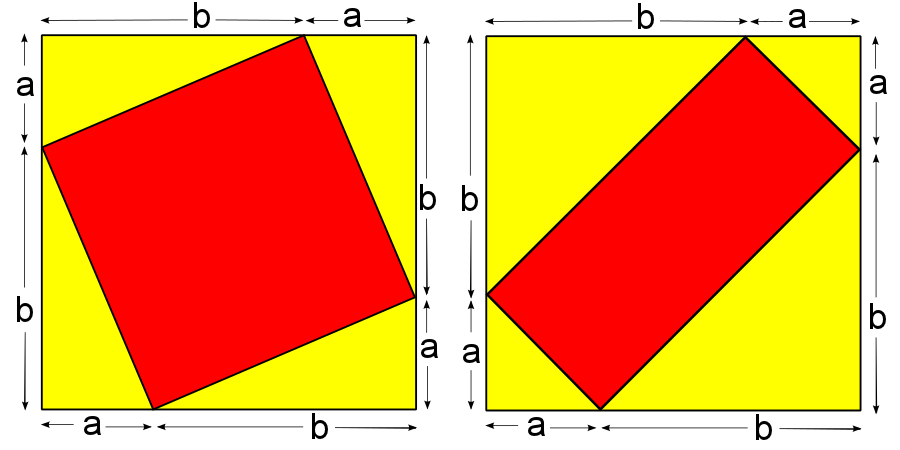#### You may also like### Kissing Triangles

Determine the total shaded area of the 'kissing triangles'.### Isosceles

Prove that a triangle with sides of length 5, 5 and 6 has the same area as a triangle with sides of length 5, 5 and 8. Find other pairs of non-congruent isosceles triangles which have equal areas.Four rods, two of length a and two of length b, are linked to form a kite. The linkage is moveable so that the angles change. What is the maximum area of the kite?

##### Age 11 to 14Challenge Level
Suppose we have a yellow square of side length $a+b$.

We can draw quadrilaterals in this square so that one vertex lies on each side of the square, and cuts each side into one segment of length $a$ and one segment of length $b$, as below:Can you prove that in each of these images the area of the red quadrilateral is exactly half the area of the yellow square?

Try to find two different ways to prove it - one algebraic, and one geometric.

Here are two more images showing quadrilaterals drawn on the yellow square.Can you prove that the areas of these two red quadrilaterals sum to the area of the yellow square?

Again, try to prove this in both an algebraic and a geometric way.

With thanks to Don Steward, whose ideas formed the basis of this problem.